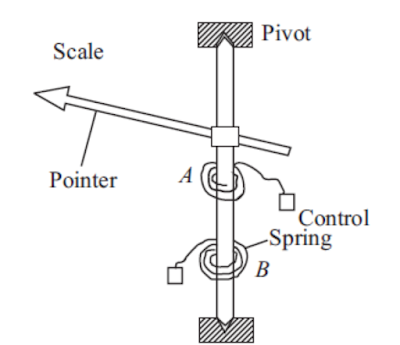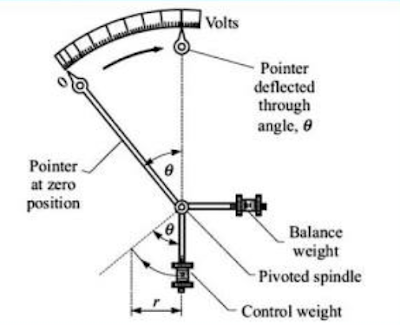# CONTROL TORQUE IN ELECTRICAL INSTRUMENT

0
138

## CONTROL TORQUE IN ELECTRICAL INSTRUMENT

### WHAT IS CONTROLLING TORQUE?

#### 1. SPRING CONTROLArrangement for Spring Control Method.

#### Design of Springs: – Usually flat springs are used because they require less space.

The controlling torque developed by the spring is equal to

Tc = {Y*b*t3*Ø}/ 12* L ;

Where,  Y = Young’s Modulus of spring material

b = width of the spring.

t = thickness of the spring.

Ø = deflection of the pointer.

L = length of the spring.

### ADVANTAGES OF SPRING CONTROL METHOD

1. The instrument can be placed in any position i.e., horizontal as well as vertical.

2. This method does not increase the weight of the instrument as springs are light.

3. Scale is uniform in this method.

### DISADVANTAGES OF SPRING CONTROL METHOD

a. Temperature variations can affect the length and design of the spring and hence also changes the controlling torque.

b. Springs get deterioted with the time and hence its accuracy is also lost.

### 2. GRAVITY CONTROL METHOD

In this method, natural downward pull due to gravity is employed. A small weight “W” is placed on the arm attached to the moving system.

At the zero position of the pointer, the control weight W is in the vertical position and therefore no controlling torque is produced. However, under the action of the deflecting force, the pointer deflects by an angle “Ø” from its zero position.
As shown in the figure below, as pointer moves weight will also move.Arrangement of gravity control method.

But due to gravity, the control weight would try to come back to its original vertical position and hence produces the necessary controlling torque. There are two components of the control weight WsinØ and WcosØ.

Only the component WsinØ will provide the necessary controlling torque and helps to retain the original position.

Thus,   Tc = WsinØ

It is seen from above equation that controlling torque is directly proportional to the sine of the deflected angle.